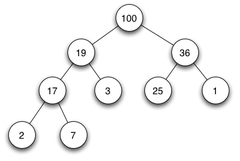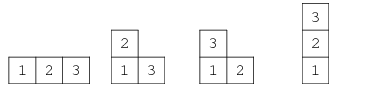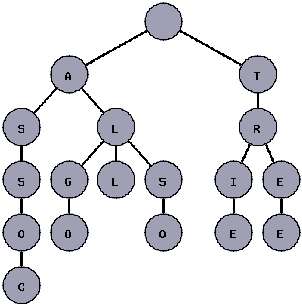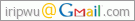# 分类: 2009 November

## 堆排序

##### 堆的定义

1. 根结点若有子树,则子树一定也是堆.
2. 根结点一定大于(或小于)子结点.

• i的父结点:Parent(i)=i/2
• i的左叶子:Left(i)=2*i
• i的右叶子:Right(i)=2*i+1##### 堆的操作

• insert: 因为需要维持堆的完全二叉树形态,需要先将节点x插到新的位置,然后把x做上升调整(元素交换)到合适的位置,即当x比它的父亲小时,把x与它的父亲交换.
• delete: 因为需要维持堆的完全二叉树形态,需要先用”最后一个节点”x把要删除的节点(根节点)覆盖掉,再把x下降到合适的位置,即当x比它的默一个儿子大时,把x和它的较小儿子交换.

insert(e)     //自底向上

while e不在根节点并且e>parent(e)

delete(data[])    //自顶向下

while 以x为根的子树不满足堆的性质

##### 堆的应用

[题目1] Dull Game (via: sicily 1778)

There are infinite balls with distinct number and distinct weight in a pool. At the beginning there is an empty box, too. Ivan and Kevin are going to play the game for N rounds. In the ith round, Ivan will take away Ai balls from the pool and put them into the box. Then Kevin will choose the first Bi heaviest balls from the box and throw them away. Too stupid, isn’t it!
What Ivan and Kevin want to know is which is the heaviest one among the remaining balls in the box after N rounds done.

[分析] 这是一道赤裸裸的堆排序,给出代码:

 ```1 2 3 4 5 6 7 8 9 10 11 12 13 14 15 16 17 18 19 20 21 22 23 24 25 26 27 28 29 30 31 32 33 34 35 36 37 38 39 40 41 42 43 44 45 46 47 48 49 50 51 52 53 54 55 56 57 58 59 60 61 62 63 64 65 66 67 68 69 70 71 72 73 74 75 76 77 78 79 80 81 82 83 84 85 ``` ```/* source code of submission 262362, Zhongshan University Online Judge System */ #include #include using namespace std; #define MAX 100000 int test,n,a,b; typedef struct node{ int num; int weight; }Node; long st_num; Node st[MAX]; bool operator <(Node a,Node b) { return a.weightst[num/2].weight) swap(num,num/2); num/=2; } } void st_delete() { int num=st_num; swap(1,num); st_num--; num=1; while(num<=st_num/2) { if(st[num*2].weight>st[num*2+1].weight) if(st[num*2].weight>st[num].weight) swap(num*2,num); else if(st[num*2].weight<=st[num*2+1].weight) if(st[num*2+1].weight>st[num].weight) swap(num*2+1,num); num*=2; } } Node st_top() { Node top; top=st; return top; } int main() { cin>>test; int i; while(test--) { st_num=0; cin>>n; priority_queuepq; while(n--) { cin>>a>>b; for(i=0;i>temp.num>>temp.weight; pq.push(temp); // st_insert(temp); } for(i=0;i

[题目2] 可怜的奶牛(via: 黑书 P90)

[分析] 此题最容易想出的方法就是简单的模拟,每天根据奶牛的产奶量找出最小值,则复杂度为O(n),总复杂度为O(T*n).其中,T为模拟的天数,因为周期不超 过10,如果有的牛永远也不会被吃掉,那么最多需要模拟2520天(2520是1,2,..10的最小公倍数) 才能确定答案,复杂度难以忍受.

##### 特殊的堆:杨式图表

• 如果格子(i,j)没有元素,则它右边和上边的相邻格子也没有元素.
• 如果格子(i,j)有元素a[i,j],则它右边和上边的相邻格子要么没有元素,要么比a[i,j]大.

1~n所组成的Young Tableau的个数可以由下式给出:

1. a(1)=1,a(2)=2
2. a(n)=a(n-1)+(n-1)a(n-2)• 插入算法(bumping算法): 从最底行开始,从左到右找底一个比x大的数.如果找不到,将x插到行尾;如果找到了符合条件的y,交换x和y,并从第二行(注意,最底层行号为1,倒数第二层行号为2,以次类推)开始用累死的方法插入y.由于行数(这里,黑书写错了)递减,所以算法必定终止.
• 查找算法: 从n*m的矩阵底行最右列开始查找.如果当前元素比x大,向左查找(因为当前列一定不存在该元素),一旦遇到比x大的元素就向上查找(因为当前行的左边不可能有该元素),直到找值等于到x的元素为止.容易知道,总的比较次数不超过m+n.

[题目3] Young Tableau问题的描述是这样的,一个由N个小方块组成的阵列(不一定要是矩形,可以是一个任意”光滑”且”单调”的组合),从1到N这N个数填入方块中,要求全部填满并且一个数只能填一个方格一次.并且满足,每个数的上方的数和左方的数比它大.求最后一共有多少种填法.比如一个4*4格子的正方形,1~16这16个数按照上述规则填入,那么一共多少种填法.

update: 未完,我体力不支了,不一定续写,thanks to the sucking net speed.

## 使用字典树–Using Tries

##### Introduction

• trie插入和查询字符串的时间复杂度是O(L)(L表示一个特定字符串的长度).这比起set可要快上不少,即便和哈希表比较,trie还是稍微更快的.
• set和哈希表只支持在dictionary中查找到希望查询的字符串完全匹配的字符串;而trie允许我们容错查找,比如说,字符不一致,共同前缀,字符丢失等等.

trie不仅在TopCoder类型的比赛中很实用,它在软件项目中也有相当多的实际应用.举个例子,考虑一下网络浏览器.你知道浏览器是如何根据已输入的文字自动填充或者给出给出候选输入的吗?是的,通过trie你可以轻易地实现这个功能.你知道拼写检查器是怎么知道你所输入的每一个单词是否在词典中的吗?答案还是一样的,通过trie.你同样可以通过trie,判断输入的单词是否在词典中存在,如果不存在的话给出一个改正的建议单词.

##### What’s a Tree?

• trie是一棵树,每一结点是一个字母或者是一个前缀.
• 树根表示一个空的字符串(“”),根的直接后继结点表示长度为1的前缀,深度为2的结点表示长度为2的前缀,深度为3的结点表示长度为3的前缀,以此类推.换句话说,深度为k的结点表示长度为k的后缀.
• 以v和w表示trie树的两个结点,v为w的直接前驱,那么v的前缀必须是w的前缀.##### Coding a Trie

trie树可以通过不同的方法构造,有些方法可以用来在字典中查找与目的字符串略微有些不同的所有其他字符串,但是有些方法仅仅支持完全匹配的查询.本文介绍的是后者.trie的实现将以伪代码的形式给出,因为不同的人使用不同的语言.

• 插入.该功能允许向词典中插入单词.
• 统计前缀.该功能统计所有以一特定字符串作前缀的单词的数目.
• 统计单词.该功能统计词典中与一给定的字符串完全匹配的单词数目.
• 这里的trie树仅仅支持英文字母表中的字符.

structure Trie

integer words;
integer prefixes;
reference edges;

initialize(vertex)
addWord(vertex, word);
integer countPrefixes(vertex, prefix);
integer countWords(vertex, word);

initialize(vertex)

vertex.words=0
vertex.prefixes=0
for i=0 to 26

edges[i]=NoEdge

addWord函数有两个参数,单词插入所在的结点,插入的单词.当一个字符串string被添加到结点vertex时,我们将根据word的首字母,将它加入相应的vertex的分支,然后将word的首字母去掉.当找不到相应的vertex分支时,我们需要对vertex结点新建一个分支.(Arthur989注:一直递归着这么做下去,直至word为空)所有TopCoder比赛支持的语言都能够在常数时间内删去一个字符串的首元素,而无需拷贝原字符串或者移动字符串的其他元素.

addWord(vertex, word)

if isEmpty(word)
vertex.words=vertex.words+1
else

vertex.prefixes=vertex.prefixes+1
k=firstCharacter(word)
if(notExists(edges[k]))

edges[k]=createEdge()
initialize(edges[k])

cutLeftmostCharacter(word)
addWord(edges[k], word)

countWords(vertex, word)

k=firstCharacter(word)
if isEmpty(word)

return vertex.words

else if notExists(edges[k])

return 0

else

cutLeftmostCharacter(word)
return countWords(edges[k], word);

countPrefixes(vertex, prefix)

k=firstCharacter(prefix)
if isEmpty(word)

return vertex.prefixes

else if notExists(edges[k])

return 0

else

cutLeftmostCharacter(prefix)
return countWords(edges[k], prefix)

##### Other Kinds of Tries

countWords(vertex, word, missingLetters)

k=firstCharacter(word)
if isEmpty(word)

return vertex.word

else if notExists(edges[k]) and missingLetters=0

return 0

else if notExists(edges[k])

cutLeftmostCharacter(word)
return countWords(vertex, word, missingLetters-1)

else

r=countWords(vertex, word, missingLetters-1)
cutLeftmostCharacter(word)
r=r+countWords(edges[k], word, missingLetters)
return r

## 无聊(1)

_________________

sudo apt-get install girlfriend

girlfriend: 依赖: house但是它将不会被安装
girlfriend: 依赖: car但是它将不会被安装
house,car: 依赖: money但是它将不会被安装
E: 无法安装的软件包

• ### Dont Ask To Ask• ### About

Hello,我是rip,这是我的个人博客,目前都是些闲言碎语的垃圾随笔,努力转型为技术博客中...--from ripwu